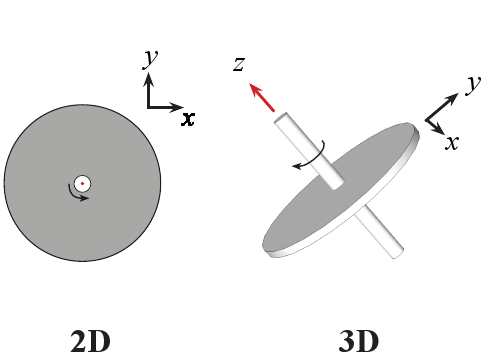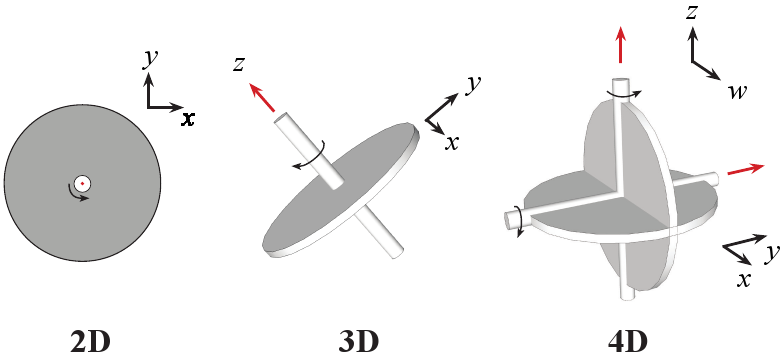# The Lost 4-Dimensional Rotation

Update: r/math discussion

Occasionally, an article about the Fourth Dimension pops up on Hacker News. For instance, The Fourth dimension: Toward a geometry of higher reality (1984) submitted February 2022.

In the spirit of the classic Flatland, such articles usually try to give an intuitive sense of higher-dimensional space through 2D or 3D analogy, eschewing a more mathematical approach. Here I’ll try to do the opposite. I’ll use a little math to show something I find very unintuitive about 4D that rarely comes up: that an object in 4D can spin around two perpendicular axes at the same time.

## No good analogy!

Here’s how I might try to explain it intuitively: imagine a car wheel spinning about its axle. In 4D you could ram a second axle long-ways through the tire, start spinning the wheel around that axis, and still drive the car smoothly down the road.

This analogy is not great – we’ll see why at the end – so let’s step through the dimensions and do some math to sort this out.

## The 2D rotation

The 2D rotation describes how a points on a plane change position when you rotate around the origin. It can be written as a 2x2 matrix that transforms point (x, y) to point (x’, y’). It’s usually written like this:

You can check your intuition by thinking about rotating a point (1, 0) by ninety degrees counter-clockwise. It should end up as (0, 1): going from pointing right along the x-axis to pointing up along the y.

## The 3D rotationThe 3D rotation is not much more complicated. The fixed point at the origin becomes a fixed line along the z-axis.

The 3D rotation is a simple extension of the 2D rotation, as long as we choose the axis of rotation to lie in the 3rd-dimension (the z-direction). The 3D rotation matrix below sends a point (x, y, z) to (x’, y’, z’), and you can see that it never moves the z-coordinate of any point.

Can you see this is just the 2D rotation with an extra dimension? If you’re describing how a record spins on a turntable – even in a 3D world – you don’t need to worry about the height of the record!

## 4D rotations

Now going to 4D, you could just extend the rotation matrix like we did for 2D -> 3D.

And indeed this is a valid 4D rotation. Instead of one fixed axis, it now has one fixed plane. If I split our 4D rotation matrix into quarters, you can see it in the bottom right-hand corner:

Since we have a whole free plane just doing nothing, couldn’t we also shove a 2D rotation in there? Turns out we can, and the following is a perfectly valid 4D rotation:

Now we have the totally new rotation! It has no precedent in 2 or 3 dimensions. We can now rotate in two different planes, through two different angles, at the same time.In the 4D rotation, you can rotate about two orthogonal planes. The axis of each rotation likes in the plane of the other. Here w denotes the new dimension.

## Conclusion

So why was our analogy of a wheel with an extra axle stuck through it unsatisfying? Because not only are the axes perpendicular, the planes of rotation are as well. That’s two axes and two planes that need to be mutually perpendicular, and you just can’t have four perpendicular vectors in 3D so our intuition – and by extension the analogy – fails.

If you want to read more about why I was getting into this back in 2008, feel free to jump to Appendix C of my doctoral dissertation to learn about the character table of the 600 cell.

Thanks to u/frustrated-nerd for pointing out an error in my original post.